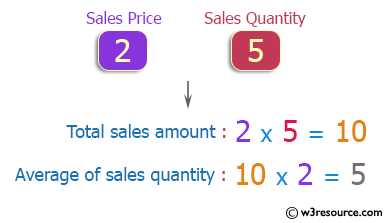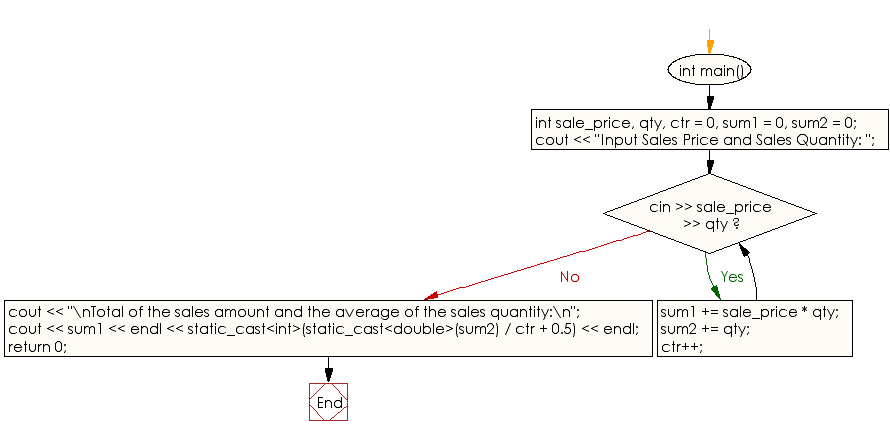﻿ C++ : Compute total sales amount, average sales quantity

# C++ Exercises: Compute total sales amount and the average sales quantity

## C++ Basic: Exercise-73 with Solution

Write a C++ program that accepts the sales unit price and sales quantity of various items and computes the total sales amount and the average sales quantity. All input values must be greater than or equal to 0 and less than or equal to 1,000. In addition, the number of pairs of sales unit and sales quantity does not exceed 100. If a fraction occurs in the average of the sales quantity, round to the first decimal place.

Pictorial Presentation:Sample Solution:

C++ Code :

``````#include <iostream>

using namespace std;

int main()
{
int sale_price, qty, ctr = 0, sum1 = 0, sum2 = 0;
cout << "Input Sales Price and Sales Quantity: ";
while (cin >> sale_price >> qty)
{
sum1 += sale_price * qty;
sum2 += qty;

ctr++;
}

cout << "\nTotal of the sales amount and the average of the sales quantity:\n";
cout << sum1 << endl << static_cast<int>(static_cast<double>(sum2) / ctr + 0.5) << endl;

return 0;
}
``````

Sample Output:

```Sample Input: 2 5
Input Sales Price and Sales Quantity:
Total of the sales amount and the average of the sales quantity:
10
5
```

Flowchart:C++ Code Editor:

What is the difficulty level of this exercise?

﻿

## C++ Programming: Tips of the Day

Why is there no std::stou?

The most pat answer would be that the C library has no corresponding "strtou", and the C++11 string functions are all just thinly veiled wrappers around the C library functions: The std::sto* functions mirror strto*, and the std::to_string functions use sprintf.

Ref: https://bit.ly/3wtz2qA

We are closing our Disqus commenting system for some maintenanace issues. You may write to us at reach[at]yahoo[dot]com or visit us at Facebook